• Python文本进度条
2021-10-13 09:35:44

文本进度条：编程通过格式化字符串输出和时间延迟实现控制台风格文本进度条绘制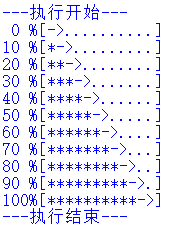效果如上图

import time
scale=10
print("执行开始".center(scale,'-'))
for i in range(scale+1):
a='*'*i
b="."*(scale-i)
c=(i/scale)*100
print("\r{:^3.0f}%[{}->{}]".format(c,a,b),end='\n')
time.sleep(0.05)
print("执行结束".center(scale,'-'))

更多相关内容
• python实现文本进度条 程序进度条 加载进度条 单行刷新功能，具体内容如下所示： 利用time库来替代某个程序 的进行过程，做实例， 思路是，简单打印出来程序进度 单行刷新关键是\r， python默认是print后换行，所以...
• Python学习案例——文本进度条

个人练习，仅供参考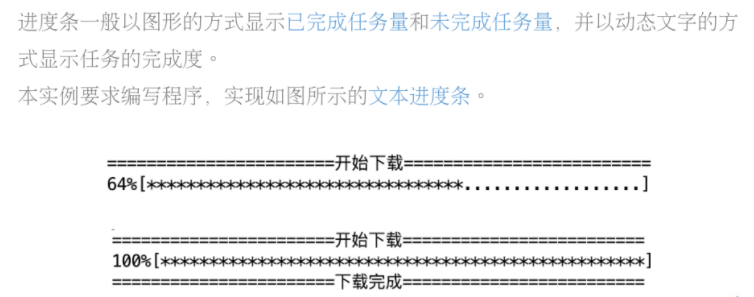这是程序要求

import time

length = 50                    #进度条长度
string1 = "开始下载"
print(string1.center(56, "=")) #将string1居中显示，然后填充=
for i in range(length + 1):
c = (i / length) * 100     #进度条
a = '*' * i                #填充*
b = '.' * (length - i)     #填充.
print("\r{:3.0f}%[{}->{}]".format(c, a, b), end="")  # \r回车，让光标回到行首
time.sleep(0.08)           # 暂停给定秒数后执行程序
string2 = "下载完成"
print("\n" + string2.center(56, "="))

这是程序代码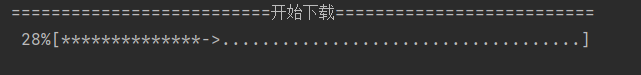这是运行过程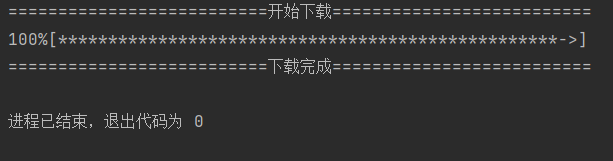这是运行结果

展开全文• 看到进度条一点点逼近百分之百，是不是很有成就感？！

看到进度条一点点逼近百分之百，是不是很有成就感？！

下面我们就借助 python 来实现它。

为了能够更直观，我们姑且赋予开始和结束提示：

#文本进度条

import time

scale=10

print('------执行开始------')

for i in range(scale+1):
a='*'*i
b='.'*(scale-i)
c=(i/scale)*100
print('{:^3.0f}%[{}->{}]'.format(c,a,b))
time.sleep(0.1)

print('------执行结束------')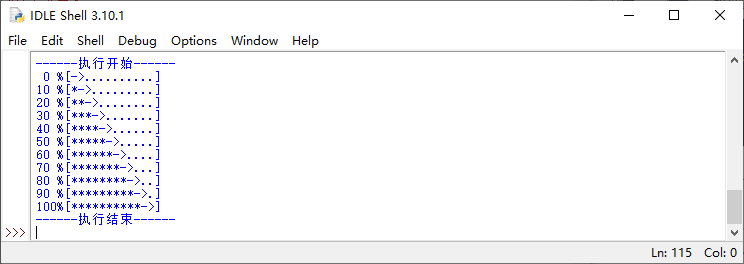或者为了体现时间逝者如斯夫，可以借助 time.perf_counter() 函数：

import time

scale=20

print('执行开始'.center(scale,'-'))

start=time.perf_counter()

for i in range(scale+1):
a='*'*i
b='.'*(scale-i)
c=(i/scale)*100
dur=time.perf_counter()-start
print('{:^3.0f}%[{}->{}]{:^6.2f}s'.format(c,a,b,dur))
time.sleep(0.1)

print('执行结束'.center(scale,'-'))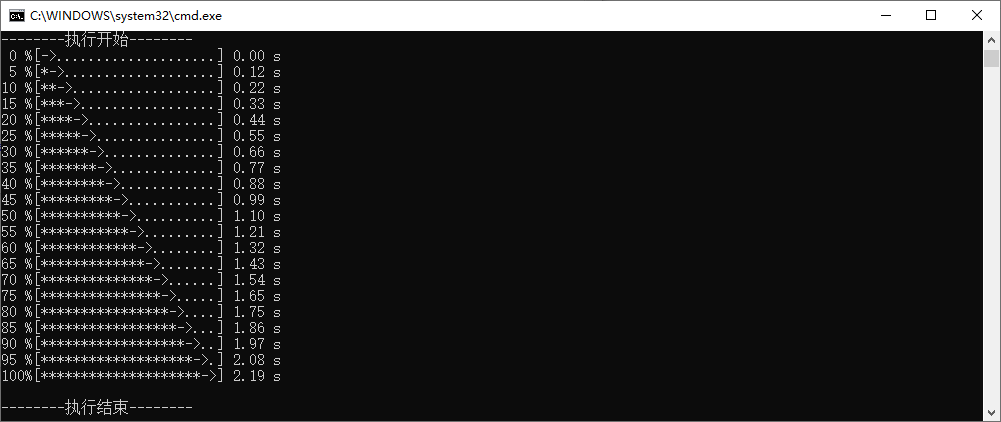要体现动感，则需要 '\r' end='' 出场：

import time

scale=100

start=time.perf_counter()

for i in range(scale+1):
a='*'*i
b='.'*(scale-i)
c=(i/scale)*100
dur=time.perf_counter()-start
print('\r{:^3.0f}%[{}->{}]{:^6.2f}s'.format(c,a,b,dur),end='')
time.sleep(0.1)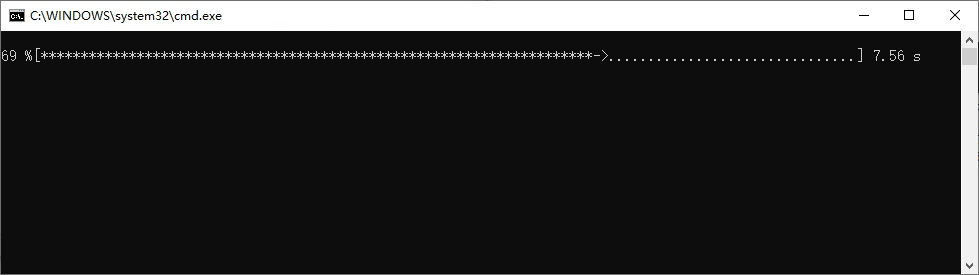怎么样，是不是很有律动感呢？

展开全文• Python文本进度条的实现和简单注释解析 需求分析： 采用字符串打印的方式，打印输出可以动态变化的文本进度条，要求进度条需要能在一行中逐渐变化。 问题分析： 如何获得文本进度条的变化时间？→采用sleep()模拟...
• #文本进度条.py import time scale = 50 print("执行开始".center(scale, "-"))//居中对齐 start = time.perf_counter()//获取起始时间 for i in range(scale+1): a = i*'*' b = (scale-i)*'.' c = (i/scale)*100...
• 文本进度条的实现 引入 import time 主要是为了使程序暂停一会，以便人眼便于观察 \r 将光标的位置回退到本行的开头位置 {：3.0f}表示以三位数据宽度的形式，有效小数位数为0的表示 end" " python在默认状态下会...

### 文本进度条的实现

引入

import time
主要是为了使程序暂停一会，以便人眼便于观察

• \r 将光标的位置回退到本行的开头位置
• {：3.0f}表示以三位数据宽度的形式，有效小数位数为0的表示
• end" " python在默认状态下会在字符串后面添加换行字符，添加end关键字后就可以使print在一行不断的刷新
import time
for i in range(50):
print("\r{:3.0f}%".format(i),end="")	//\r将光标的位置回退到本行的开头位置
time.sleep(0.1)


进一步可以实现一个文本进度条式的显示

import time
scale = 100
print("执行开始".center(scale+10,"-"))
start = time.perf_counter()
for i in range(scale + 1):
a = "*" * i
b = "." * (scale - i)
c = (i/scale)*100
dur = time.perf_counter() - start
print("\r{:3.0f}%[{}-->{}]{:.2f}s".format(c,a,b,dur),end="")
time.sleep(0.1)
print("\n"+"执行结束".center(scale+10,"-"))


输出结果展开全文• 利用time库来替代某个程序 的进行过程，做实例，思路是，简单打印出来程序进度 import time tm=10 print('{:-^18}'.format('开始')) for i in range(tm+1): a='#'*i b='.'*(tm-i) c=(i/tm)*100 print('{...
• 文本进度条 实例完整效果 代码如下： #TextProBarV3.py import time scale = 50 print("执行开始".center(scale//2, "-")) start = time.perf_counter() for i in range(scale+1): a = '*' * i b = '.' * ...
• Python实现简单的文本进度条，且单行动态刷新经验分享
• 简单的文本进度条代码解析引入time库打印一行作为开始最后也打印一个结束的标签定义变量等于10，文本进度条大概的宽度是10使用for循环来模拟进度，for i in range()能够不断地产生循环每次答应都会显示当前的休眠...
• ## python语言程序设计——文本进度条

千次阅读 多人点赞 2019-10-30 10:23:40
如今的文本打开需要读取进度，而进度条的动画效果其实也可以用python来实现，我们先来看示例一： import time scale = 10 print('------执行开始------') for i in range(scale+1): a = '*' * i b = '.' * (scale-...
• 怎么利用格式化输出和时间延迟来实现控制台风格的文本进度条
• 1.0代码：import time#引入time库scale=10#文本进度条宽度print("------执行开始------")for i in range(scale+1):#模拟一个进度a='*'*i#字符串被复制的次数，"*"表示百分比所表达的信息b='.'*(scale-i)c=(i/scale)*...
• Python实例4：文本进度条采用字符串方式打印动态变化的文本进度条；进度条需要在一行中逐渐变化；采用sleep()模拟一个持续的进度；单行动态刷新：用后打印的字符覆盖之前的字符；print()需要被控制；打印后光标退回...
• python实现文本进度条 程序进度条 加载进度条 单行刷新版功能，详细内容如下所示：利用time库来替代某个程序 的进行过程，做实例，思路是，简单打印出来程序进度单行刷新版关键是\r，python默认是print后换行，所以...
• 经过自己一段时间的学习，已经略有...**文本进度条相信大家都玩过游戏吧，面对加载的游戏数据，我们可能会遇到这样一种情况：网络卡顿的时候，进度条缓缓移动却迟迟不能加载完全，实在是消磨人们的耐心（狗头）在...
• #文本进度条 scale=10 print("执行开始".center(scale,"-")) for i in range(scale+1): a='*'*i b='.'*(scale-i) c=(i/scale)*100 print('{:^3.0f}%[{}->{}]'.format(c,a,b)) time.sleep(0.1) print('执行...倒计时
• 前言 文本进度条是什么？...# 本程序的目的是做一个文本进度条 # 注意：这玩意在IDLE里是看不出效果，因为IDLE把\r给屏蔽了，可以在命令行中执行 import time scale = 50 print("执行开始".centershell
• （2）其中scale定义的是文本进度条的长度在学习中我们把它赋值为10 （3）print()输出格式 （4）其中运用到了for i in range():循环，在循环末尾通过format函数，将其内的值赋予槽中。 单行动态刷新： 1. （1）用后...
• 文本进度条 采用字符串方式打印可以动态变化的文本进度条 进度条需要能在一行中逐渐变化 单行动态刷新 刷新的本质：用之后打印的字符覆盖之前的字符 不能换行：print()需要被控制 要能回退：打印后光标退回到......